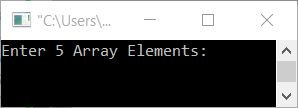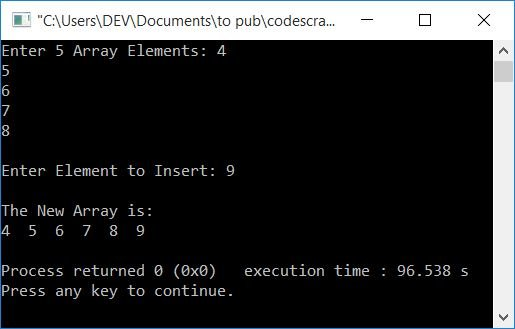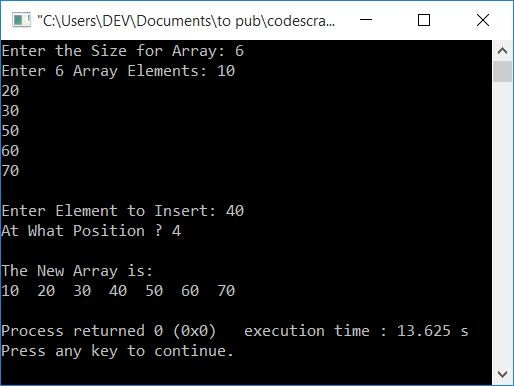# C++ Program to Insert an Element in an Array

In this article, you will learn and get code to insert an element into an array in the C++ language. Here is the list of programs available in this article:

## Add an Element to the End of an Array

This program prompts the user to enter 5 numbers or elements for an array, followed by the element to insert at the end of the given array.

The question is: write a program in C++ to insert an element at the end of an array. Here is its answer:

```#include<iostream>
using namespace std;
int main()
{
int arr, i, elem;
cout<<"Enter 5 Array Elements: ";
for(i=0; i<5; i++)
cin>>arr[i];
cout<<"\nEnter Element to Insert: ";
cin>>elem;
arr[i] = elem;
cout<<"\nThe New Array is:\n";
for(i=0; i<6; i++)
cout<<arr[i]<<"  ";
cout<<endl;
return 0;
}```

This program was built and runs under the Code::Blocks IDE. Here is its initial output:Now supply five inputs as five numbers or elements for the array, say 4, 5, 6, 7, and 8, and then enter a number or element, say 9, to insert in the array. Here is the sample output produced by the above program after providing these inputs:This program receives 5 array elements using a for loop, one by one. That is, if the user enters 5 array elements as 4, 5, 6, 7, and 8, then these elements get stored in arr[] in the following way:

• arr=4
• arr=5
• arr=6
• arr=7
• arr=8

After receiving all 5 elements for the array, the value of i is now 5. So just receive another input, which is the element to insert, and initialise it to arr[i] or arr. Finally, print the new array.

## Insert an Array Element at a Specific Position

To insert an element in an array in C++ programming, you have to ask the user to enter the size and elements of the array. And then ask to enter the element to insert and at what position, as shown in the program given below:

After inserting the element at the desired position, don't forget to display the new array on the screen.

```#include<iostream>
using namespace std;
int main()
{
int arr, i, elem, pos, tot;
cout<<"Enter the Size for Array: ";
cin>>tot;
cout<<"Enter "<<tot<<" Array Elements: ";
for(i=0; i<tot; i++)
cin>>arr[i];
cout<<"\nEnter Element to Insert: ";
cin>>elem;
cout<<"At What Position ? ";
cin>>pos;
for(i=tot; i>=pos; i--)
arr[i] = arr[i-1];
arr[i] = elem;
tot++;
cout<<"\nThe New Array is:\n";
for(i=0; i<tot; i++)
cout<<arr[i]<<"  ";
cout<<endl;
return 0;
}```

Here is its sample run with the following user input:

• 6 as the array size
• 10, 20, 30, 50, 60, and 70 as 6 array elements
• 40 as the element to insert
• 4 as the position to insert the element.

After supplying these inputs, here is the snapshot that shows all the operations that have been done on the output screen:In the above program, with the help of pos's value (the desired position where the user wants to insert the new element), we've shifted all the elements from this position to one index forward. After doing this task, the previous element in this position gets moved to its next position. Therefore, we're free to initialize the new element at this position.

Don't forget to increment the value of tot (the size of the array) after inserting the new element into it. Now print the new array that contains the newly inserted element at the desired or specific position, as shown in the output given above.

For example, the dry run of the following block of code

```for(i=tot; i>=pos; i--)
arr[i] = arr[i-1];
arr[i] = elem;```

With the same user input as in the preceding sample run, it goes as follows:

• Here are the values before program flow comes to this block of code:
• tot = 6
• arr = 10, arr = 20, arr = 30, arr = 50, arr = 60, and arr = 70.
• elem = 40
• pos = 4
• Now the execution of this small block of code starts with a for loop.
• That is, its initialization part executes at first, but only once. So the value of tot gets initialized to i. Therefore, i=6
• Now the condition i>=pos or 6>=4 evaluates to be true, therefore program flow goes inside the loop.
• Inside the loop, arr[i-1] or arr[6-1] or arr or 70 gets initialized to arr[i] or arr. So arr=70. As you can see, the number at the last index (the fifth index) is transferred to the fourth index (the sixth index).
• Now the program flow goes to the update part of the for loop, which decrements the value of i (so i = 5) and evaluates the condition again.
• That is the condition i>=pos or 5>=4 evaluates to be true again, so program flow returns to the loop.
• This process continues, until the condition of for loop evaluates to be false. Before its condition evaluated to be false, here are the new values of arr[]:
• arr = 70
• arr = 60
• arr = 50
• Now the element or number 50, which was present in the fourth position (third index), gets moved to the fifth position (fourth index). Because indexing begins at 0, we consider the element at the fourth index to be the element in the fifth position.
• Therefore, the fourth position (third index) is free to initialize any new element.
• So just initialize the new element on it. Therefore, the value of elem, which is 40, gets initialized to arr[i] or arr.

#### Same Program in Other Languages

C++ Online Test

« Previous Program Next Program »